## Rolles and Langranges Theorem vsaq’s

Rolles and Langranges Theorem

## Rolles and Langranges Theorem vsaq’s

### Rolle’s theorem

If f: [a, b] ⟶ R be a function satisfying the  following conditions

• f is continuous on [a, b]
• f is differentiable (a, b)
• f(a) = f(b)

then there exists at least one cϵ (a, b) such that f’(c) = 0

### Langrage’s theorem

If f: [a, b] ⟶ R be a function satisfying the following conditions

• f is continuous on [a, b]
• f is differentiable (a, b)

then there exists at least one cϵ (a, b) such that f’(c) =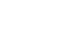Rolles and Langranges Theorem vsaq’s

###### 1. State Rolle’s theorem

Sol:

If f: [a, b] ⟶ R be a function satisfying the following conditions

• f is continuous on [a, b]
• f is differentiable (a, b)
• f(a) = f(b)

then there exists at least one cϵ (a, b) such that f’(c) = 0

###### 2. State Langrage’s theorem

Sol: If f: [a, b] ⟶ R be a function satisfying the following conditions

• f is continuous on [a, b]
• f is differentiable (a, b)

then there exists at least one cϵ (a, b) such that f’(c) =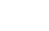###### in (1, 3) such that f’ (c) = 0

Sol: Given function is f(x) = (x – 1) (x – 2) (x – 3)

(i)f(x) is continuous on [1, 3]

(ii) f(x) is differentiable on (1, 3)

(iii) f(1) = (1 – 1) (1 – 2) (1 – 3)

= 0 (– 1)( – 2)

= 0

f(3) = (3 – 1) (3 – 2) (3 – 3)

= (2) (1) (0)

= 0

f(1) = f(3)

f’(x) = (1 – 0) (x – 2) (x – 3) + (x – 1) (1 – 0) (x – 3) + (x – 1) (x – 2) (1 – 0)

= (x – 2) (x – 3) + (x – 1) (x – 3) + (x – 1) (x – 2)

= x2 – 3x – 2x + 6 + x2 – 3x – x + 3 + x2 – 2x – x + 2

= 3x2 – 12x + 11

f’ (c) = 3c2 – 12c + 11

by Rolle’s Theorem f’ (c ) = 0

3c2 – 12c + 11 = 0###### y = f(x) = x2  + 4 on [– 3, 3]

Sol:  Given function is f(x) = (x – 1) (x – 2) (x – 3)

f(x) is continuous on [– 3, 3]

f(x) is differentiable on (– 3, 3)

f (– 3) = (– 3)2 + 4 = 9 + 4 = 13

f (3) = (3)2 + 4 = 9 + 4 = 13

f (– 3) =  f(3)

By Rolle’s theorem there exist c ∈ (– 3, 3) such that f’ (c) = 0

f’ (x) = 2x

f’ (c) = 2c

⟹ 2c = 0

C = 0 ∈ (– 3, 3)

###### 5. Find the value of ‘c’ from Rolle’s theorem for the function f(x) = x2 – 1 on [– 1, 1]

Sol: Given function is  f(x) = x2 – 1

f(x) is continuous on [– 1, 1]

f(x) is differentiable on (– 1, 1)

f (– 1) = (– 1)2 – 1 = 1 – 1 = 0

f (– 1) = (– 1)2 – 1 = 1 – 1 = 0

f (– 1) =  f(1)

By Rolle’s theorem there exist c ∈ (– 1, 1) such that f’ (c) = 0

f’ (x) = 2x

f’ (c) = 2c

⟹ 2c = 0

c = 0 ∈ (– 1, 1)

6. It is given that Rolle’s theorem holds for the function f(x) = x3 + bx2 + ax on [1, 3] with c = 2 +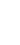. Find the values of a and b.

Sol:  Given function is f(x) = x3 + bx2 + ax on [1, 3] satisfying Rolle’s theorem

⟹ f(x) is continuous on [ 1, 3]

f(x) is differentiable on (1, 3)

f (1) = f (3)

and there exists at least one c = 2 +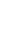ϵ (1, 3) such that f’ (c) = 0

now f(1) = f(3)

1 + b + a = 27 + 9b + 3a

2a + 8b + 26 = 0

a + 4b + 13 = 0 ———- (1)

f’ (x) = 3 x2 + 2b x + a

f’ (c) = 0

⟹ 3 c2 + 2bc + a  = 0

Since  c = 2 +3  + 2b ( ) + a = 0

3  + 4b ) + a = 0

3  + 4b ) + a = 0

+  + 4b +  + a = 0

13 +  + 4b +  + a = 0 ———- (2)

Equation (2) – Equation (1)from (1) a + 4(– 6) + 13 = 0

a – 24 + 13 = 0

a = 11

∴ a = 11, b = – 6

###### 7. Verify Rolle’s theorem for the function f(x) = sin x – sin 2x on [0, π]

Sol: Given function is f(x) = sin x – sin 2x

f(x) is continuous on [0, π]

f(x) is differentiable on (0, π)

f(0) = sin (0) – sin 2(0) = 0

f( π) = sin ( π) – sin 2(π) = 0

f(0) = f( π)

now f’ (x) = cos x – 2 cos 2x

f ‘ (c) = 0

cos c – 2 cos 2c = 0

cos c – 2(2 cos2 c – 1) = 0

cos c – 4 cos2 c – 2 = 0

4 cos2 c – cos c + 2 = 0c =  ∈ (0, π)

∴ Rolle’s theorem is verified

###### 8. Verify Rolle’s theorem for the function f(x) = (x2 – 1) (x – 2) on [ – 1, 2]

Sol:  Given function is f(x) = (x2 – 1) (x – 2)

f(x) is continuous on [ – 1, 2]

f(x) is differentiable on (– 1, 2)

f (– 1) = (1 – 1) (– 1 – 2) = 0 (– 3) = 0

f (2) = (4 – 1) (2 – 2) = 3 (0) = 0

f (– 1) = f (2)

f(x) satisfies all the conditions of Rolle’s theorem

f’ (x) = (x2 – 1) (1 – 0)   + (2x – 0) (x – 2)

f’ (x) = (x2 – 1) (1)   + (2x) (x – 2)

f’ (x) = x2 – 1 + 2x2 – 4x

= 3x2 – 4x – 1

f’ (c) = 0

3c2 – 4c – 1 = 0∴ Rolle’s theorem is verified

9. Verify Rolle’s theorem for the function f(x) = x (x + 3)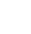on [ – 3, 0]

Sol:  Given function is f(x) = x (x + 3)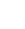f(x) is continuous on [ – 3, 0]

f(x) is differentiable on (– 3, 0)

f (– 3) = (– 3) (– 3 + 3)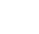= (– 3) (0)= 0

f (0) = (0) (0 + 3)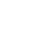= 0

f (– 3) = f (0)

f(x) satisfies all the conditions of Rolle’s theorem

f’ (x) = 1 (x + 3)   + x (1 + 0)    + x (x + 3)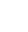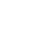f’ (c) = 0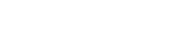c2 – c – 6 = 0

c2 – 3c + 2c – 6 = 0

c (c – 3) + 2 (c – 3) = 0

(c – 3) (c + 2) = 0

c = 3 or c = – 2

c = 3 ∉(– 3, 0) and c =  – 2  ∈ (– 3, 0)

∴ Rolle’s theorem is verified

###### 10. Show that there is no real number ‘k’ for which the equation x2 – 3x + k has two distinct roots in [0, 1].

Sol: given function is f (x) = x2 – 3x + k

Let α, β be the two distinct roots of f (x) and 0 < α < β <1

f’ (α) = 0 and f’ (β) = 0

f(x) is continuous on [ α, β]

f(x) is differentiable on (α, β)

f(α) = f(β)

f’ (x) = 2x – 3

f’ ( c ) = 0

2c – 3 = 0

2c = 3

c = 3/2

###### 11. Verify Rolle’s theorem for the function f(x) = log (x2 + 2) – log 3  on [ – 1, 1]

Sol: Given function is f(x) = log (x2 + 2) – log 3

f(x) is continuous on [ – 1, 1]

f(x) is differentiable on (– 1, 1)

f(– 1) = log (1 + 2) – log 3 = log 3 – log 3 = 0

f(1) = log (1 + 2) – log 3 = log 3 – log 3 = 0

f(– 1) = f(1)

f(x) satisfies all the conditions of Rolle’s theorem

f’ (x) =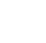(2x) =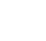by Rolle’s theorem f’ (c) = 0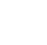= 0 ⟹ 2c = 0 ⟹ c = 0∈ (– 1, 1)

∴ Rolle’s theorem is verified

###### 13. Find ‘c’ so that f’ (c) =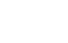; f(x) = ex, a =0, b = 1

Sol: Given f(x) = ex, a =0, b = 1

f (b) = f(1) = e

f (a) = f(0) = e0 = 1

f’ (x) = ex

f’ (c) =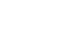ec =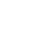ec = e – 1

c =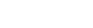###### 14. Verify Lagrange’s Mean value theorem for the function f(x) = x2 – 1 on [2, 3]

Sol: Given function is  f(x) = x2 – 1

f(x) is continuous on [ 2, 3]

f(x) is differentiable on (2, 3)

f’(x) = 2x

By Lagrange’s mean value theorem f’ (c) =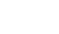2c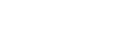= 5

c = 5/2 ∈  (2, 3)

∴ Lagrange’s Mean value theorem is verified

###### 15. Verify the Lagrange’s Mean value theorem for the function f(x) = sin x – sin 2x on [0, π]

Sol: Given function is  f(x) = sin x – sin 2x

f(x) is continuous on [0, π]

f(x) is differentiable on (0, π)

f(π) = sin π – sin 2 π = 0 – 0 = 0

f(0) = sin 0 – sin 2(0) = 0 – 0  = 0

f’(x) = cos x – 2cos 2x

By Lagrange’s mean value theorem f’ (c) =cos c – 2cos 2c =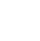= 0

cos c – 2cos 2c = 0

cos c – 2 (2 cos2c – 1) = 0

cos c – 4cos2c + 2 = 0

4 cos2 c – cos c – 2 = 0

cos c =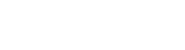=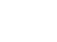c = (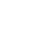)∈ (2, 3)

∴ Lagrange’s Mean value theorem is verified

16. Verify Lagrange’s Mean value theorem for the function f(x) = log x on [1, 2]

Sol:  Given function is f(x) =  log x

f(x) is continuous on [1, 2]

f(x) is differentiable on (1, 2)

f(2) = log 2; f(1) = log 1 = 0

f’ (x) =

By Lagrange’s mean value theorem f’ (c) =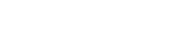1/c = log 2

c =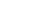∈ (1, 2)

∴ Lagrange’s Mean value theorem is verified

17. Find the point on the graph of the curve y = x3 where the tangent is parallel to the chord joining the points (1, 1) and (3, 27)

Sol: Given curve is y = x3

f’ (x) = 3x2

let A = (1, 1) and B = (3, 27)

slope of AB=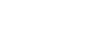= 13

given slope of AB = slope of the tangent

3x2 = 13∴ the point is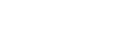##### Visit my Youtube Channel: Click on Below Logo